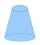# Cone - problems

1. Masquerade ballMarie wants to make a cone-shaped witch's hat for a masquerade ball. How much material will it need if it counts on an annular rim with diameters of 28cm and 44cm? Hat side length is 30cm. Add 5% of the material to the bust. Round to cm2.
2. Castle towerThe castle tower has a cone-shaped roof with a diameter of 10 meters and a height of 8 meters. Calculate how much m² of coverage is needed to cover it if we must add one-third for the overlap.
3. The diagram 2The diagram shows a cone with slant height 10.5cm. If the curved surface area of the cone is 115.5 cm2. Calculate correct to 3 significant figures: *Base Radius *Height *Volume of the cone
4. Pile of sandA large pile of sand has been dumped into a conical pile in a warehouse. The slant height of the pile is 20 feet. The diameter of the base of the sand pile is 31 feet. Find the volume of the pile of sand.
5. Axial sectionAxial section of the cone is equilateral triangle with area 208 dm2. Calculate volume of the cone.
6. Cone A2VSurface of cone in the plane is a circular arc with central angle of 126° and area 415 cm2. Calculate the volume of a cone.
7. ConeCalculate volume and surface area of ​​the cone with diameter of the base d = 15 cm and side of cone with the base has angle 52°.
8. ConeCircular cone of height 15 cm and volume 5699 cm3 is at one-third of the height (measured from the bottom) cut by a plane parallel to the base. Calculate the radius and circumference of the circular cut.
9. Sand pileAuto sprinkled with sand to approximately conical shape. Workers wanted to determine the volume (amount of sand) and therefore measure the circumference of the base and the length of both sides of the cone (over the top). What is the volume of the sand c
10. RotationRight triangle with legs 14 cm and 20 cm rotate around longer leg. Calculate the volume and surface area of the formed cone.
11. CapJesters hat is shaped a rotating cone. Calculate how much paper is needed to the cap 60 cm high when head circumference is 52 cm.
12. Rotary coneThe volume of the rotation of the cone is 472 cm3 and angle between the side of the cone and base angle is 70°. Calculate lateral surface area of this cone.
13. ConeCircular cone with height h = 29 dm and base radius r = 3 dm slice plane parallel to the base. Calculate distance of the cone vertex from this plane, if solids have the same volume.
14. TowerHow many m2 of copper plate should be to replace roof of the tower conical shape with diameter 24 m and the angle at the vertex of the axial section is 144°?
15. CanopyMr Peter has metal roof cone shape with a height of 127 cm and radius 130 cm over well. He needs paint the roof with anticorrosion. How many kg of color must he buy if the manufacturer specifies the consumption of 1 kg to 3.3 m2?
16. Rotary coneRotary cone whose height is equal to the circumference of the base, has a volume 229 cm3. Calculate the radius of the base circle and height of the cone.
17. 2x coneCircular cone height 76 cm was cut plane parallel with base. Volume of these two small cones is the same. Calculate the height of the smaller cone.
18. Cut and coneCalculate the volume of the rotation cone which lateral surface is circle arc with radius 15 cm and central angle 63 degrees.
19. Rotating coneCalculate volume of a rotating cone with base radius r=12 cm and height h=7 cm.
20. Truncated coneCalculate the height of the rotating truncated cone with volume V = 1115 cm3 and a base radii r1 = 7.9 cm and r2 = 9.7 cm.

Do you have an interesting mathematical problem that you can't solve it? Enter it, and we can try to solve it.

To this e-mail address, we will reply solution; solved examples are also published here. Please enter e-mail correctly and check whether you don't have a full mailbox.